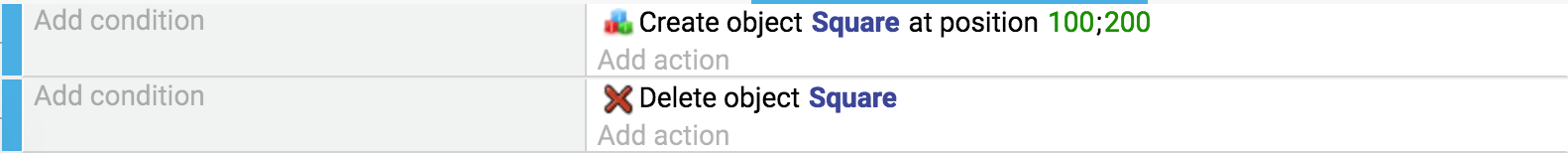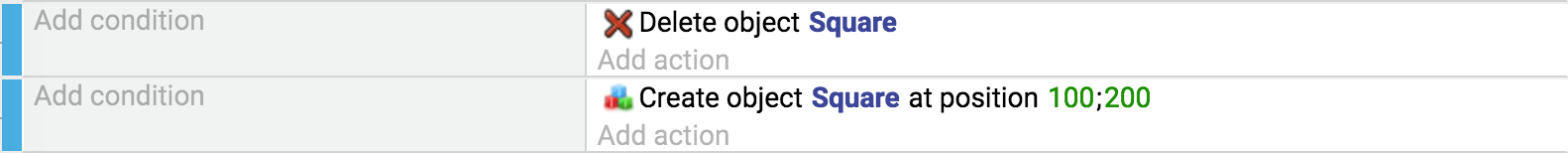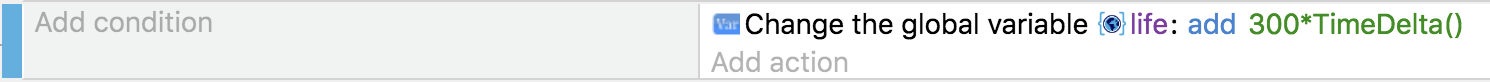# Basic game making concepts: What you need to know to start with GDevelop

The basic concepts of GDevelop are simple. Read the following information to get started with the software. If you don't have GDevelop, download the latest version here!

## Coordinates

Objects on the GDevelop screen/scene have an X and a Y coordinate. These coordinates correspond to the horizontal position (X-axis) and the vertical position (Y-axis) on the Cartesian plane.

The X-coordinate decreases as you scroll to the left and increases as you scroll to the right. The Y-coordinate decreases as you scroll upwards and increases as you scroll downwards.

In order to move or rotate objects, you will need to specify the desired angle in degrees. The illustration below demonstrates how GDevelop understands the angle of rotation:

If you use trigonometric functions like the sine or the cosine, you will need to express the angles in radians. (1 radian = 180/PI degrees and 1 degree = PI/180 radians. PI is the number which is approximately equal to 3.14159).

## Events and how objects are picked

Events, that you use to create the rules of your game, are composed of conditions and actions.

Most conditions and actions refer to objects. Conditions run a test on the objects. Actions manipulate the object.

An action, without a condition, refers to all objects. If you use an object for the first time in an event, GDevelop will test or modify all objects with this name in the scene. If you use the object in the same event again, GDevelop will test or modify only the objects which have been picked by the previous conditions.

This process is an application of logic. For example, this event has no condition. It will delete all objects called “Square”:The event below has a condition. It will delete only the “Square” objects with an X position that is less than 100 pixels:See it in action! 🎮Open this example online: https://editor.gdevelop-app.com/?project=example://object-selection

## Events: the order is important

The order of events is critical!

Events at the top are executed first. Events are executed every time your game is displayed. This display is called a frame. It executes about 60 times per second. The following examples are not equivalent:• The first pair of events actions creates a “Square” object at coordinates X: 100. Y: 200 (100;200). Then the event deletes the created square immediately. No “Square” is displayed on the screen. It's deleted just after being created.
• The second pair of events actions deletes all of the “Square” objects from the scene/screen. It then creates one “Square” at the scene/screen coordinates X: 100, Y: 200 (100;200). This event shows a square is visible on the scene (before being deleted at the next frame. It will, then, be recreated immediately.

## Moving objects using built-in forces

Moving objects can be achieved with forces. Forces are used to “push” objects.

You can specify:

• the Cartesian coordinates of a force (i.e. X and Y, in pixels).
• its polar coordinates (the force vector angle and length).
• the force damping, a coefficient between 0 and 1.

Each second, the force-length will be multiplied by the dispersion: a value of 1 means that force will continue forever. A value of 0 will make it stop immediately. All intermediate values will cause the force to gradually stop.

Some behaviors that you can add to your objects will move the objects by themselves. This negates the need to use forces. In this case see below:

### Example

Say you want to move an object towards the bottom:

You can add a force using X/Y coordinates by specifying 0 for the X coordinate and, say, 150 pixels for the Y coordinate. You can also use polar coordinates and add a force with an angle of 90° and a length of 150 pixels.

### Other ways of moving objects

Some behaviors, like the Physics engine or Pathfinding, may move the objects by themselves. In this case, it is better not to use the builtin forces system and instead rely only on actions provided by the extensions used.

## Variables

Variables allow you to store data. For example, a variable can store a number or a text. We might compare them to drawers or boxes where we can file notes. Any data can be stored in a variable, as long as it is in text or number format. Such things as the number of lives a player has remaining, a player's high-score, the number of bullets left, and/or the number of enemies killed are all examples of what can be stored in a variable. You are probably going to store numbers in variables as a common practice.

Actions and conditions test or change a variable value.

#### Where are variables stored? (variable "scope")

The scope of a variable determines the place a variable can be accessed. In GDevelop, there are three variable scopes are available:

• Global variables are accessible from all the game scenes. For instance, they can be used to store the player's score across different levels/scenes.
• Scene variables are only accessible from the scene they are created in. They can be used for data that only concerns one scene. A Scene variable would be able to access the time remaining to complete the level/scene.
• Object variables only concern one object. For example, a hero can have a “Health” or “Ammo” variable.
Read more about variables on the Variables page. Variables are used a lot and you can see examples of all you can use them in most examples and in the tutorials.
Want to see how variables are used in a tutorial video? Check this tutorial Variables explained for Non-programmers.

## Time elapsed since last frame (TimeDelta)

The game evaluates events and repaints the screen several times per second: we say that the game is refreshed, updated during a “tick”. How often this happens depends on your computer resources: a slow computer can render 25 frames per second, a fast one 60. GDevelop can usually make sure that this value is the same on all computers. However, the speed of some operations can change from computer to computer, for example, mathematical operations and the rendering of movements.

To make the speed of changes constant, use the TimeDelta() expression, which returns the time in seconds since the last frame. For example, don't do this:But do this instead:• The first event is adding 20 to the variable every time the game is refreshed (i.e. as much as possible, up to 60 times per second). It's not correct to use such event as the speed of the increase of the variable will not be the same from computer to computer: we cannot predict the value of the variable after 10 seconds for example - as it depends on the number of frames of the game painted.
• The second event is correct and reliable : The number 300 is multiplied by TimeDelta(). Thus, the variable will be increased at the same time on all computers. As TimeDelta() returns a time in second, it allows you to quantify exactly the amount: In our case, the variable will grow at 300 units/second. Thus, we can predicate that the life will increase by 3000 units in 10 seconds - whatever the number of frames painted during this time.

Remember: Use TimeDelta() when you want to continually add some amount to a value. If your action is meant to be launched only once (for example, during a collision where you then delete the object), it's ok to use a discrete value without TimeDelta - as it does not depend upon the speed of the game.

When you're moving an object using forces, there is no need to use TimeDelta() as GDevelop automatically uses it.

## And... that's almost all you need to know

➡️ You can continue to read the tutorials to know more about creating real games with GDevelop!